## 17. 电话号码的字母组合UPDATE: 2020.8.9

## 131. 分割回文串

4ms，93%，其实就是暴力回溯，还是挺简单的，一开始忘了`remove()`，直接把所有结果打出来了， 然后一直在想怎么调整递归的结构。DFS 基本的套路都忘了😂

## 60. 第 k 个排列

1. `"123"`
2. `"132"`
3. `"213"`
4. `"231"`
5. `"312"`
6. `"321"`

• 给定 n 的范围是 [1, 9]。
• 给定 k 的范围是 [1, n!]。

**示例 1: **

## 5374. 长度为 n 的开心字符串中字典序第 k 小的字符串

• 仅包含小写字母 [‘a’, ‘b’, ‘c’].
• 对所有在 1 到 s.length - 1 之间的 i ，满足 s[i] != s[i + 1] （字符串的下标从 1 开始）。

• `1 <= n <= 10`
• `1 <= k <= 100`

## 1286. 字母组合迭代器

• 一个构造函数，输入参数包括：一个 有序且字符唯一 的字符串 characters（该字符串只包含小写英文字母）和一个数字 combinationLength 。
• 函数 next() ，按 字典序 返回长度为 combinationLength 的下一个字母组合。
• 函数 hasNext() ，只有存在长度为 combinationLength 的下一个字母组合时，才返回 True；否则，返回 False。

• 1 <= combinationLength <= characters.length <= 15
• 每组测试数据最多包含 10^4 次函数调用。
• 题目保证每次调用函数 next 时都存在下一个字母组合。

## 39. 组合总和

• 所有数字（包括 target）都是正整数。

• 解集不能包含重复的组合。

## 40. 组合总和 II

candidates 中的每个数字在每个组合中只能使用一次。

• 所有数字（包括目标数）都是正整数。
• 解集不能包含重复的组合。

• 所有数字都是正整数。
• 解集不能包含重复的组合。

## 78. 子集

Update: 2020.6.20

BFS，类似于二叉树层次遍历，首先初始化一个空的 list，后面每次迭代都将 list 中的所有元素都取出来加上当前元素，再重新加入到 list 中

## 90. 子集 II

Update: 2020.6.20

## 89. 格雷编码## 526. 优美的排列

1. 第 i 位的数字能被 i 整除
2. i 能被第 i 位上的数字整除

• S 的长度不超过 12。
• S 仅由数字和字母组成。

## 1079. 活字印刷

1. `1 <= tiles.length <= 7`
2. `tiles` 由大写英文字母组成

### Bug 警告！！！## 1291. 顺次数

• 10 <= low <= high <= 10^9

## 22. 括号生成## 306. 累加数

• 你如何处理一个溢出的过大的整数输入？

## 842. 将数组拆分成斐波那契序列

• 0 <= F[i] <= 2^31 - 1，（也就是说，每个整数都符合 32 位有符号整数类型）；
• F.length >= 3；
• 对于所有的 0 <= i < F.length - 2，都有 `F[i] + F[i+1] = F[i+2]` 成立。

• `1 <= S.length <= 200`
• 字符串 S 中只含有数字。

## 139. 单词拆分

• 拆分时可以重复使用字典中的单词。
• 你可以假设字典中没有重复的单词。

BFS

## 140. 单词拆分 II

• 分隔时可以重复使用字典中的单词。
• 你可以假设字典中没有重复的单词。

UPDATE: 2020.7.8

## 473. 火柴拼正方形

1. 给定的火柴长度和在 `0``10^9`之间。
2. 火柴数组的长度不超过 15。

## 698. 划分为 k 个相等的子集

• `1 <= k <= len(nums) <= 16`
• `0 < nums[i] < 10000`

473. 火柴拼正方形其实只是这道题的一个子问题，关键搞懂这题就行了

`index==-1`为什么就直接返回 true 了？简单推一下就行了

## 491. 递增子序列

Difficulty: 中等

1. 给定数组的长度不会超过 15。
2. 数组中的整数范围是 [-100,100]。
3. 给定数组中可能包含重复数字，相等的数字应该被视为递增的一种情况。

## 679. 24 点游戏

Difficulty: 困难

1. 除法运算符 `/` 表示实数除法，而不是整数除法。例如 4 / (1 - 2/3) = 12 。
2. 每个运算符对两个数进行运算。特别是我们不能用 `-` 作为一元运算符。例如，`[1, 1, 1, 1]` 作为输入时，表达式 `-1 - 1 - 1 - 1` 是不允许的。
3. 你不能将数字连接在一起。例如，输入为 `[1, 2, 1, 2]` 时，不能写成 12 + 12 。

## 5526. 最多可达成的换楼请求数目

Difficulty: 困难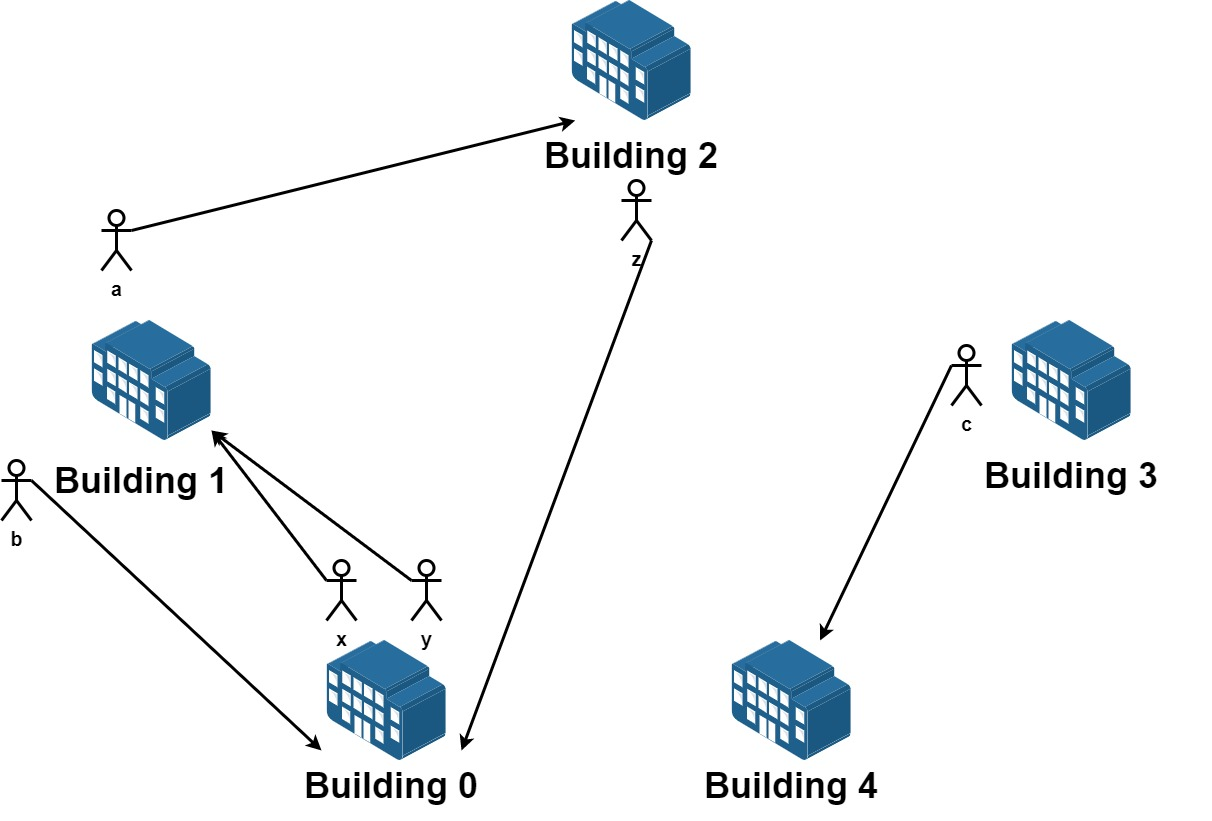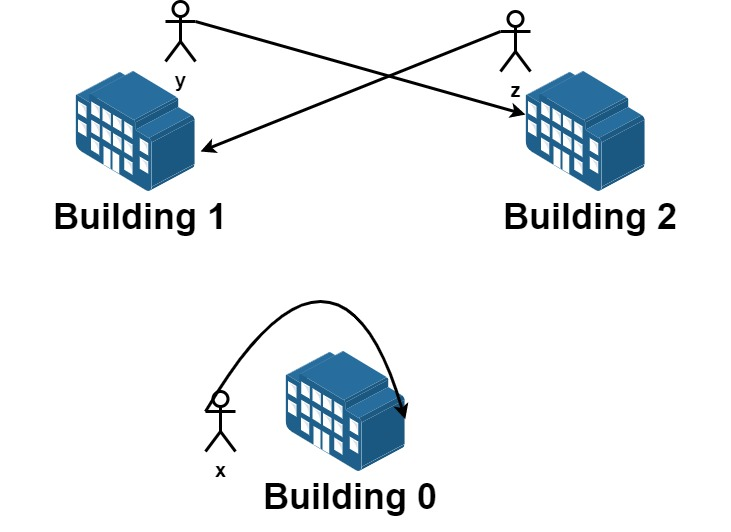• 1 <= n <= 20
• 1 <= requests.length <= 16
• requests[i].length == 2
• 0 <= fromi, toi < n

208 周赛 T4，有点白给，想好怎么处理就很简单（好不容易 T4 这么简单，被 T2，T3 给干懵了）

## 130. 被围绕的区域

21ms，8% 乍一看好像没啥问题，但是为啥这么慢呢？看看今天下午重新做的解

2ms，比较明显的区别就是 dfs 给 visit 数组赋值的时机不同，一个是在循环里面，一个是在函数开头，也就是说第一种解法并不会立即给边缘的`'O'` 标记，这样导致的问题就是它后续的节点仍然会搜索到这个边缘的 `'O'` 这样就会造成重复的计算，所以说，一定要捋清楚这个过程，不能乱写

## 417. 太平洋大西洋水流问题

1. 输出坐标的顺序不重要

2. m 和 n 都小于 150

6ms，96%，还是比较 ok 的

## 51. N 皇后

n 皇后问题研究的是如何将 n 个皇后放置在 n×n 的棋盘上，并且使皇后彼此之间不能相互攻击Hard 题，N 皇后问题可以说是很经典的问题了，之前有看过，但是都是一脸懵逼，无从下手，这一次看了下提示还是自己给做出来了

## 37. 解数独Note:

• 给定的数独序列只包含数字 `1-9` 和字符 `'.'`
• 你可以假设给定的数独只有唯一解。
• 给定数独永远是 `9x9` 形式的。

## 529. 扫雷游戏

1. 如果一个地雷（’M’）被挖出，游戏就结束了- 把它改为 ‘X’。
2. 如果一个没有相邻地雷的空方块（’E’）被挖出，修改它为（’B’），并且所有和其相邻的方块都应该被递归地揭露。
3. 如果一个至少与一个地雷相邻的空方块（’E’）被挖出，修改它为数字（’1’到’8’），表示相邻地雷的数量。
4. 如果在此次点击中，若无更多方块可被揭露，则返回面板。

Explanation: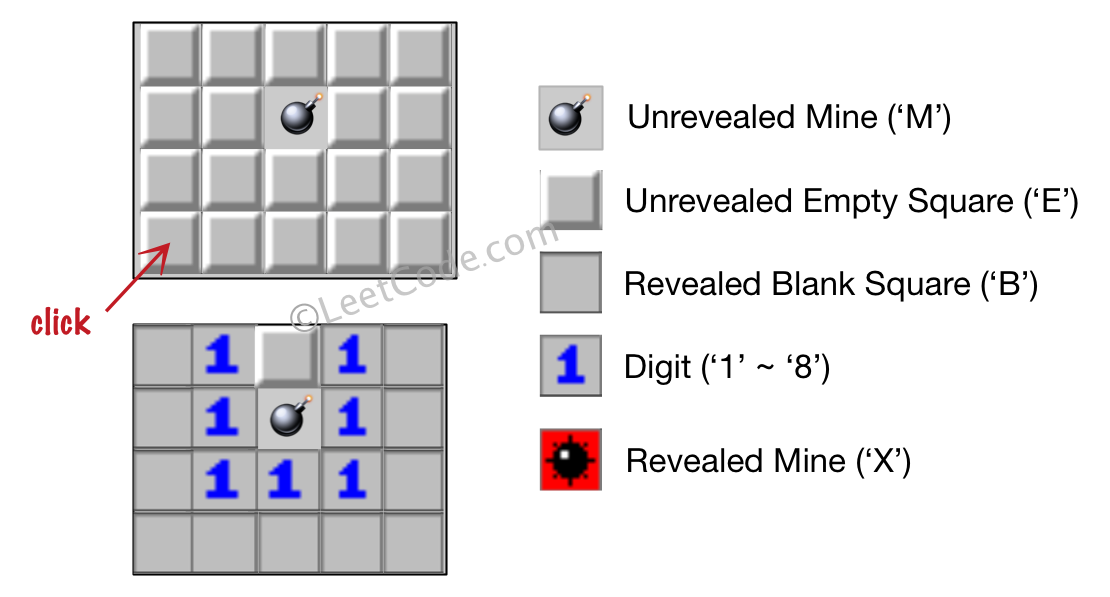Explanation:## 547. 朋友圈

• N 在 [1,200] 的范围内。
• 对于所有学生，有 M[i] [i] = 1。
• 如果有 M[i] [j] = 1，则有 M[j] [i] = 1。

## 1219. 黄金矿工

• 每当矿工进入一个单元，就会收集该单元格中的所有黄金。
• 矿工每次可以从当前位置向上下左右四个方向走。
• 每个单元格只能被开采（进入）一次
• 不得开采（进入）黄金数目为 0 的单元格。
• 矿工可以从网格中 任意一个 有黄金的单元格出发或者是停止。

• -`1 <= grid.length, grid[i].length <= 15`
• `0 <= grid[i][j] <= 100`
• 最多 25 个单元格中有黄金。

## 面试题 13. 机器人的运动范围

• `1 <= n,m <= 100`
• `0 <= k <= 20`

## 1020. 飞地的数量

1. `1 <= A.length <= 500`
2. `1 <= A[i].length <= 500`
3. `0 <= A[i][j] <= 1`
4. 所有行的大小都相同

dfs 搜索就完事儿了，水题

## 934. 最短的桥

1. `1 <= A.length = A.length <= 100`
2. `A[i][j] == 0``A[i][j] == 1`

## 733. 图像渲染

Difficulty: 简单

• `image` 和 `image` 的长度在范围 `[1, 50]` 内。
• 给出的初始点将满足 `0 <= sr < image.length` 和 `0 <= sc < image.length`
• `image[i][j]` 和 `newColor` 表示的颜色值在范围 `[0, 65535]`内。

## 1559. 二维网格图中探测环

Difficulty: 困难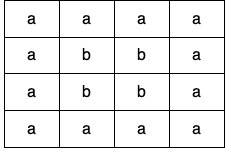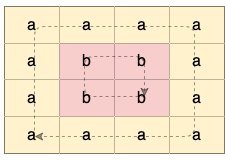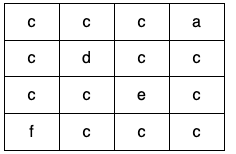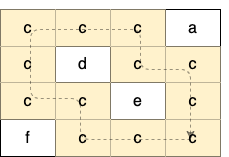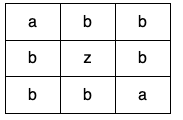• `m == grid.length`
• `n == grid[i].length`
• `1 <= m <= 500`
• `1 <= n <= 500`
• `grid` 只包含小写英文字母。

33 双周赛的 T4，总体不是很难，dfs 或者并查集都可以

## 332. 重新安排行程

Difficulty: 中等

1. 如果存在多种有效的行程，请你按字符自然排序返回最小的行程组合。例如，行程 [“JFK”, “LGA”] 与 [“JFK”, “LGB”] 相比就更小，排序更靠前
2. 所有的机场都用三个大写字母表示（机场代码）。
3. 假定所有机票至少存在一种合理的行程。
4. 所有的机票必须都用一次 且 只能用一次。

## 841. 钥匙和房间

Difficulty: 中等

`N` 个房间，开始时你位于 `0` 号房间。每个房间有不同的号码：`0，1，2，...，N-1`，并且房间里可能有一些钥匙能使你进入下一个房间。

1. `1 <= rooms.length <= 1000`
2. `0 <= rooms[i].length <= 1000`
3. 所有房间中的钥匙数量总计不超过 `3000`

Difficulty: 中等